login  home  contents  what's new  discussion  bug reports help  links  subscribe  changes  refresh  edit

# Edit detail for SandBoxFrobeniusAlgebra revision 24 of 26

 1 2 3 4 5 6 7 8 9 10 11 12 13 14 15 16 17 18 19 20 21 22 23 24 25 26 Editor: Bill Page Time: 2011/02/18 09:08:03 GMT-8 Note: associativity implies turtle is snail, jacobi implies snails vanish

changed:
-viewed as an operator with two inputs $i,j$ and one
viewed as a linear operator with two inputs $i,j$ and one

changed:
-\begin{axiom}
-in?(JP,ideal ravel YY)  -- associative
-in?(JP,ideal ravel YC)  -- commutative
-in?(JP,ideal ravel YA)  -- anti-commutative
-in?(JP,ideal ravel YX) -- Jacobi identity
-\end{axiom}

Consider the ideals of the associator, commutator, anti-commutator
and Jacobi identity
\begin{axiom}
YYI:=ideal ravel YY;
in?(JP,YYI)  -- associative
YCI:=ideal ravel YC;
in?(JP,YCI)  -- commutative
YAI:=ideal ravel YA;
in?(JP,YAI)  -- anti-commutative
YXI:=ideal ravel YX;
in?(JP,YXI) -- Jacobi identity
\end{axiom}

Three traces of two graftings of an algebra gives six
(2,0)-forms.

changed:
-
Left and right deer forms are identical but different from snails.

changed:
-\end{axiom}
test(RD=RS)
test(RD=LS)
\end{axiom}

changed:
-
The turles are symmetric
\begin{axiom}
test(RT=reindex(RT,[2,1])
test(LT=reindex(LT,[2,1])
\end{axiom}
Five of the six forms are independent.
\begin{axiom}
test(RT=RS)
test(RT=LS)
test(RT=RD)
test(LT=RS)
test(LT=LS)
test(LT=RD)
\end{axiom}
Associativity implies right turtle equals right snail
and left turtle equals left snail.
\begin{axiom}
in?(ideal ravel(RT-RS),YYI)
in?(ideal ravel(LT-LS),YYI)
\end{axiom}
If the Jacobi identity holds then both snails are zero
\begin{axiom}
in?(ideal ravel(RS),YXI)
in?(ideal ravel(LS),YXI)
\end{axiom}
and right turtle and deer have opposite signs
\begin{axiom}
in?(ideal ravel(RT+RD),YXI)
\end{axiom}


References

An n-dimensional algebra is represented by a (2,1)-tensor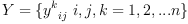viewed as a linear operator with two inputs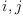and one output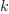. For example in 2 dimensions

axiom
n:=2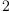(1)
Type: PositiveInteger?
axiom
T:=CartesianTensor(1,n,FRAC POLY INT)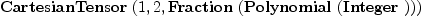(2)
Type: Domain
axiom
Y:T := unravel(concat concat
[[[script(y,[[i,j],[k]])
for i in 1..n]
for j in 1..n]
for k in 1..n]
)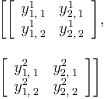(3)
Type: CartesianTensor?(1,2,Fraction(Polynomial(Integer)))

Given two vectors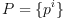and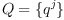axiom
P:T := unravel([script(p,[[],[i]]) for i in 1..n])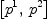(4)
Type: CartesianTensor?(1,2,Fraction(Polynomial(Integer)))
axiom
Q:T := unravel([script(q,[[],[i]]) for i in 1..n])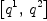(5)
Type: CartesianTensor?(1,2,Fraction(Polynomial(Integer)))

the tensor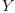operates on their tensor product to yield a vector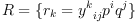axiom
R:=contract(contract(Y,3,product(P,Q),1),2,3)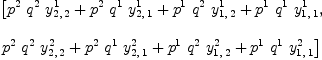(6)
Type: CartesianTensor?(1,2,Fraction(Polynomial(Integer)))

Pictorially:

  P Q
Y
R

or more explicitly

Pi Qj
\/
\
Rk


In Axiom we may use the more convenient tensor inner product denoted by * that combines tensor product with a contraction on the last index of the first tensor and the first index of the second tensor.

axiom
R:=(Y*P)*Q(7)
Type: CartesianTensor?(1,2,Fraction(Polynomial(Integer)))

An algebra is said to be associative if:

  Y    =    Y
Y       Y


Note: the right hand side of the equation above is implicitly the mirror image of the left hand side:

  i   j   k   i  j     k   i     j  k
\  |  /     \/     /     \     \/
\ | /       \    /       \    /
\|/    =    e  k    -    i  e
|           \/           \/
|            \           /
l             l         l


This requires that the following (3,1)-tensor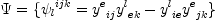(8)
(associator) is zero.
axiom
YY := reindex(reindex(Y,[1,3,2])*reindex(Y,[1,3,2]),[1,4,3,2])-Y*Y; ravel(YY)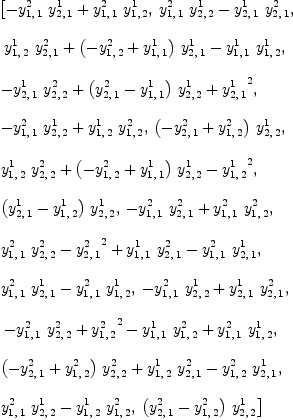(9)
Type: List(Fraction(Polynomial(Integer)))

The algebrais commutative if:

  Y = Y

i   j     i  j     j  i
\ /   =   \/   -   \/
|         \       /
k          k     k


This requires that the following (2,1)-tensor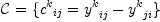(10)
(commutator) is zero.
axiom
YC:=Y-reindex(Y,[1,3,2])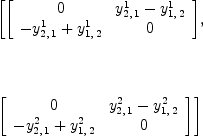(11)
Type: CartesianTensor?(1,2,Fraction(Polynomial(Integer)))

A basis for the ideal defined by the coefficients of the commutator is given by:

axiom
groebner(ravel(YC))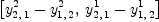(12)
Type: List(Polynomial(Integer))

The algebrais anti-commutative if:

  Y = -Y

i   j     i  j     j  i
\ /   =   \/   =   \/
|         \       /
k          k     k


This requires that the following (2,1)-tensor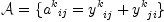(13)
(anti-commutator) is zero.
axiom
YA:=Y+reindex(Y,[1,3,2])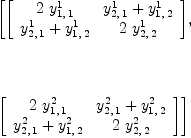(14)
Type: CartesianTensor?(1,2,Fraction(Polynomial(Integer)))

A basis for the ideal defined by the coefficients of the commutator is given by:

axiom
groebner(ravel(YA))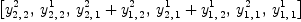(15)
Type: List(Polynomial(Integer))

The Jacobi identity is:

            X
Y =  Y + Y
Y  Y     Y

i     j     k  i      j     k  i     j      k   i  j   k
\    |    /    \    /     /    \     \    /     \  \ /
\   |   /      \  /     /      \     \  /       \  0
\  |  /        \/     /        \     \/         \/ \
\ | /          \    /          \    /           \  \
\|/     =      e  k      -     i  e       -     e  j
|              \/              \/               \/
|               \              /                /
l                l            l                 l


An algebra satisfies the Jacobi identity if and only if the following (3,1)-tensor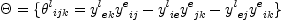(16)
is zero.

axiom
YX := YY - reindex(contract(Y,1,Y,2),[3,1,4,2]); ravel(YX)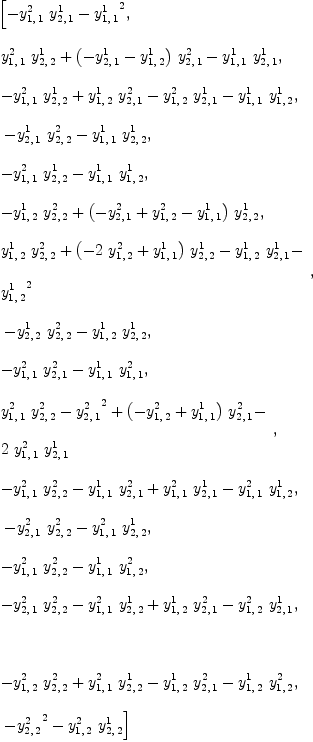(17)
Type: List(Fraction(Polynomial(Integer)))

A scalar product is denoted by the (2,0)-tensor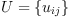axiom
U:T := unravel(concat
[[script(u,[[],[j,i]])
for i in 1..n]
for j in 1..n]
)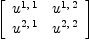(18)
Type: CartesianTensor?(1,2,Fraction(Polynomial(Integer)))

## Definition 1

We say that the scalar product is associative if the tensor equation holds:

    Y   =   Y
U     U


In other words, if the (3,0)-tensor:

    i  j  k   i  j  k   i  j  k
\ | /     \/  /     \  \/
\|/   =   \ /   -   \ /
0         0         0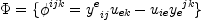(19)
(three-point function) is zero.

axiom
YU := reindex(reindex(U,[2,1])*reindex(Y,[1,3,2]),[3,2,1])-U*Y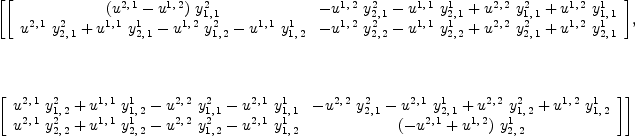(20)
Type: CartesianTensor?(1,2,Fraction(Polynomial(Integer)))

## Definition 2

An algebra with a non-degenerate associative scalar product is called pre-Frobenius.

We may consider the problem where multiplication Y is given, and look for all associative scalar products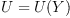or we may consider an scalar product U as given, and look for all algebras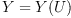such that the scalar product is associative.

This problem can be solved using linear algebra.

axiom
)expose MCALCFN
MultiVariableCalculusFunctions is now explicitly exposed in frame
initial
K := jacobian(ravel(YU),concat(map(variables,ravel(Y)))::List Symbol);
Type: Matrix(Fraction(Polynomial(Integer)))
axiom
yy := transpose matrix [concat(map(variables,ravel(Y)))::List Symbol];
Type: Matrix(Polynomial(Integer))
axiom
K::OutputForm * yy::OutputForm = 0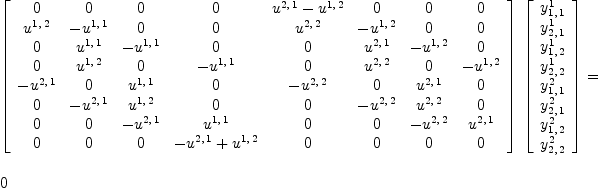(21)
Type: Equation(OutputForm?)

The matrix K transforms the coefficients of the tensorinto coefficients of the tensor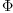. We are looking for coefficients of the tensor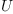such that K transforms the tensorinto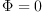for any.

A necessary condition for the equation to have a non-trivial solution is that the matrix K be degenerate.

## Theorem 1

All 2-dimensional pre-Frobenius algebras are symmetric.

Proof: Consider the determinant of the matrix K above.

axiom
Kd := factor(determinant(K)::DMP(concat map(variables,ravel(U)),FRAC INT))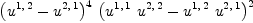(22)
Type: Factored(DistributedMultivariatePolynomial?([*002u11,*002u12,*002u21,*002u22],Fraction(Integer)))

The scalar product must also be non-degenerate

axiom
Ud:DMP(concat map(variables,ravel(U)),FRAC INT) := determinant [[U[i,j] for j in 1..n] for i in 1..n]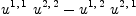(23)
Type: DistributedMultivariatePolynomial?([*002u11,*002u12,*002u21,*002u22],Fraction(Integer))

therefore U must be symmetric.

axiom
nthFactor(Kd,1)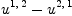(24)
Type: DistributedMultivariatePolynomial?([*002u11,*002u12,*002u21,*002u22],Fraction(Integer))
axiom
US:T := unravel(map(x+->subst(x,U[2,1]=U[1,2]),ravel U))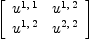(25)
Type: CartesianTensor?(1,2,Fraction(Polynomial(Integer)))

## Theorem 2

All 2-dimensional algebras with associative scalar product are commutative.

Proof: The basis of the null space of the symmetric K matrix are all symmetric

axiom
YUS:T :=  reindex(reindex(US,[2,1])*reindex(Y,[1,3,2]),[3,2,1])-US*Y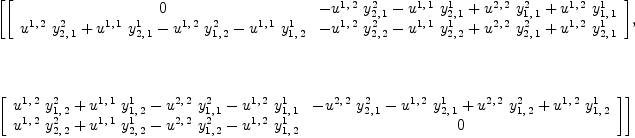(26)
Type: CartesianTensor?(1,2,Fraction(Polynomial(Integer)))
axiom
KS := jacobian(ravel(YUS),concat(map(variables,ravel(Y)))::List Symbol);
Type: Matrix(Fraction(Polynomial(Integer)))
axiom
NS:=nullSpace(KS)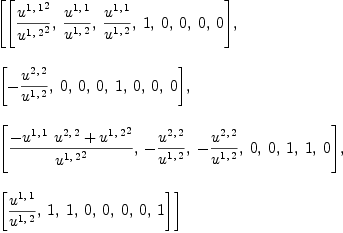(27)
Type: List(Vector(Fraction(Polynomial(Integer))))
axiom
SS:=map((x,y)+->x=y,concat map(variables,ravel Y),
entries reduce(+,[p[i]*NS.i for i in 1..#NS]))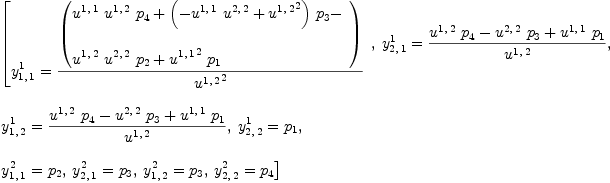(28)
Type: List(Equation(Fraction(Polynomial(Integer))))
axiom
YS:T := unravel(map(x+->subst(x,SS),ravel Y))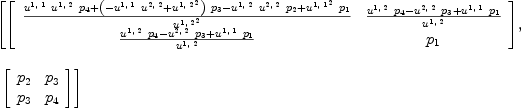(29)
Type: CartesianTensor?(1,2,Fraction(Polynomial(Integer)))

This defines a 4-parameter family of 2-d pre-Frobenius algebras

axiom
test(unravel(map(x+->subst(x,SS),ravel YUS))\$T=0*YU)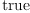(30)
Type: Boolean

Alternatively we may consider

axiom
J := jacobian(ravel(YU),concat(map(variables,ravel(U)))::List Symbol);
Type: Matrix(Fraction(Polynomial(Integer)))
axiom
uu := transpose matrix [concat(map(variables,ravel(U)))::List Symbol];
Type: Matrix(Polynomial(Integer))
axiom
J::OutputForm * uu::OutputForm = 0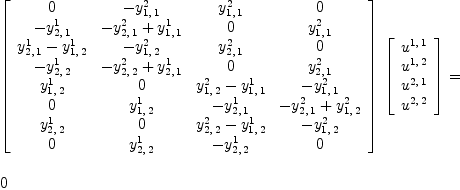(31)
Type: Equation(OutputForm?)

The matrix J transforms the coefficients of the tensorinto coefficients of the tensor. We are looking for coefficients of the tensorsuch that J transforms the tensorintofor any.

A necessary condition for the equation to have a non-trivial solution is that all 70 of the 4x4 sub-matrices of J are degenerate. To this end we can form the polynomial ideal of the determinants of these sub-matrices.

axiom
JP:=ideal concat concat concat
[[[[ determinant(
matrix([row(J,i1),row(J,i2),row(J,i3),row(J,i4)]))
for i4 in (i3+1)..maxRowIndex(J) ]
for i3 in (i2+1)..(maxRowIndex(J)-1) ]
for i2 in (i1+1)..(maxRowIndex(J)-2) ]
for i1 in minRowIndex(J)..(maxRowIndex(J)-3) ];
Type: PolynomialIdeals?(Fraction(Integer),IndexedExponents?(Symbol),Symbol,Polynomial(Fraction(Integer)))
axiom
#generators(%)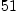(32)
Type: PositiveInteger?

## Theorem 3

If a 2-d algebra is associative, commutative, anti-commutative or if it satisfies the Jacobi identity then it is a pre-Frobenius algebra.

Proof

Consider the ideals of the associator, commutator, anti-commutator and Jacobi identity

axiom
YYI:=ideal ravel YY;
Type: PolynomialIdeals?(Fraction(Integer),IndexedExponents?(Symbol),Symbol,Polynomial(Fraction(Integer)))
axiom
in?(JP,YYI)  -- associative(33)
Type: Boolean
axiom
YCI:=ideal ravel YC;
Type: PolynomialIdeals?(Fraction(Integer),IndexedExponents?(Symbol),Symbol,Polynomial(Fraction(Integer)))
axiom
in?(JP,YCI)  -- commutative(34)
Type: Boolean
axiom
YAI:=ideal ravel YA;
Type: PolynomialIdeals?(Fraction(Integer),IndexedExponents?(Symbol),Symbol,Polynomial(Fraction(Integer)))
axiom
in?(JP,YAI)  -- anti-commutative(35)
Type: Boolean
axiom
YXI:=ideal ravel YX;
Type: PolynomialIdeals?(Fraction(Integer),IndexedExponents?(Symbol),Symbol,Polynomial(Fraction(Integer)))
axiom
in?(JP,YXI) -- Jacobi identity(36)
Type: Boolean

Y-forms

Three traces of two graftings of an algebra gives six (2,0)-forms.

Left snail and right snail:

  LS                    RS

Y /\                    /\ Y
Y  )                  (  Y
\/                    \/

i  j                        j  i
\/                          \/
\    /\              /\    /
e  f  \            /  f  e
\/    \          /    \/
\    /          \    /
f  /            \  f
\/              \/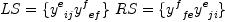(37)

axiom
LS:=contract(Y*Y,1,2)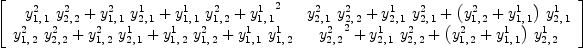(38)
Type: CartesianTensor?(1,2,Fraction(Polynomial(Integer)))
axiom
RS:=reindex(contract(reindex(Y,[1,3,2])*reindex(Y,[1,3,2]),1,2),[2,1])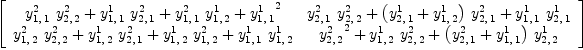(39)
Type: CartesianTensor?(1,2,Fraction(Polynomial(Integer)))
axiom
test(LS=RS)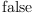(40)
Type: Boolean

Left and right deer:

   RD                 LD

\ /\/              \/\ /
Y /\              /\ Y
Y  )            (  Y
\/              \/

i            j    i            j
\    /\    /      \    /\    /
\  f  \  /        \  /  f  /
\/    \/          \/    \/
\    /\          /\    /
e  /  \        /  \  e
\/    \      /    \/
\    /      \    /
f  /        \  f
\/          \/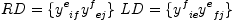(41)
Left and right deer forms are identical but different from snails.
axiom
RD:=contract(Y*Y,1,3)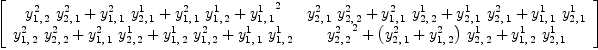(42)
Type: CartesianTensor?(1,2,Fraction(Polynomial(Integer)))
axiom
LD:=reindex(contract(reindex(Y,[1,3,2])*reindex(Y,[1,3,2]),1,3),[2,1])(43)
Type: CartesianTensor?(1,2,Fraction(Polynomial(Integer)))
axiom
test(LD=RD)(44)
Type: Boolean
axiom
test(RD=RS)(45)
Type: Boolean
axiom
test(RD=LS)(46)
Type: Boolean

Left and right turtles:

  RT                   LT

/\ / /               \ \ /\
(  Y /                 \ Y  )
\  Y                   Y  /
\/                     \/

i     j      i     j
/\    /     /        \     \    /\
/  f  /     /          \     \  f  \
/    \/     /            \     \/    \
\     \    /              \    /     /
\     e  /                \  e     /
\     \/                  \/     /
\    /                    \    /
\  f                      f  /
\/                        \/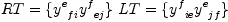(47)

axiom
RT:=contract(Y*Y,1,4)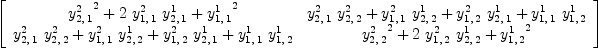(48)
Type: CartesianTensor?(1,2,Fraction(Polynomial(Integer)))
axiom
LT:=reindex(contract(reindex(Y,[1,3,2])*reindex(Y,[1,3,2]),1,4),[2,1])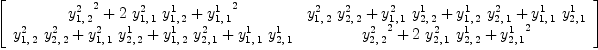(49)
Type: CartesianTensor?(1,2,Fraction(Polynomial(Integer)))
axiom
test(LT=RT)(50)
Type: Boolean

The turles are symmetric

axiom
test(RT=reindex(RT,[2,1])
Line   1: test(RT=reindex(RT,[2,1])
....A...................B
Error  A: Missing mate.
Error  B: syntax error at top level
Error  B: Possibly missing a )
3 error(s) parsing
test(LT=reindex(LT,[2,1])
Line   2: test(LT=reindex(LT,[2,1])
....A...................B
Error  A: Missing mate.
Error  B: syntax error at top level
Error  B: Possibly missing a )
3 error(s) parsing

Five of the six forms are independent.

axiom
test(RT=RS)(51)
Type: Boolean
axiom
test(RT=LS)(52)
Type: Boolean
axiom
test(RT=RD)(53)
Type: Boolean
axiom
test(LT=RS)(54)
Type: Boolean
axiom
test(LT=LS)(55)
Type: Boolean
axiom
test(LT=RD)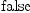(56)
Type: Boolean

Associativity implies right turtle equals right snail and left turtle equals left snail.

axiom
in?(ideal ravel(RT-RS),YYI)(57)
Type: Boolean
axiom
in?(ideal ravel(LT-LS),YYI)(58)
Type: Boolean

If the Jacobi identity holds then both snails are zero

axiom
in?(ideal ravel(RS),YXI)(59)
Type: Boolean
axiom
in?(ideal ravel(LS),YXI)(60)
Type: Boolean

and right turtle and deer have opposite signs

axiom
in?(ideal ravel(RT+RD),YXI)(61)
Type: Boolean